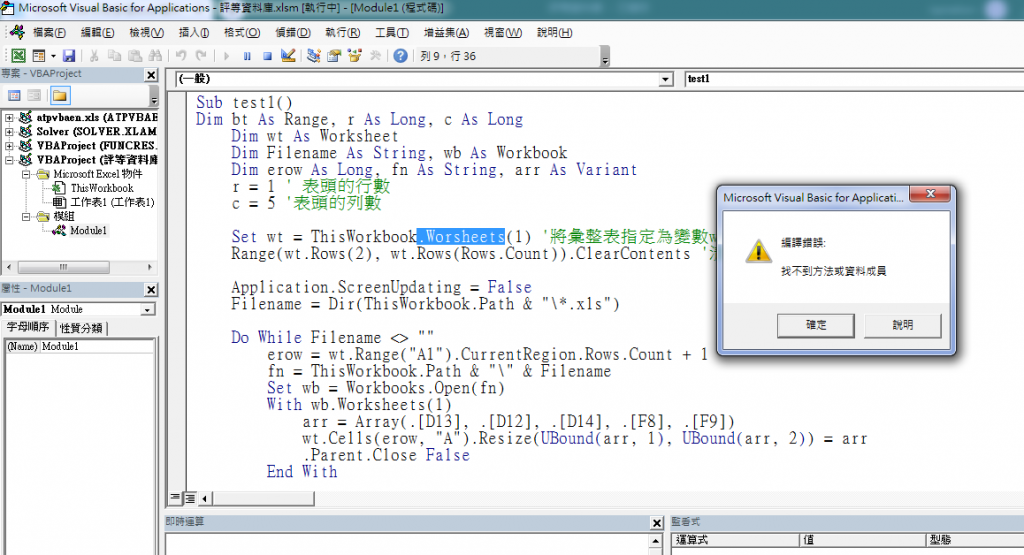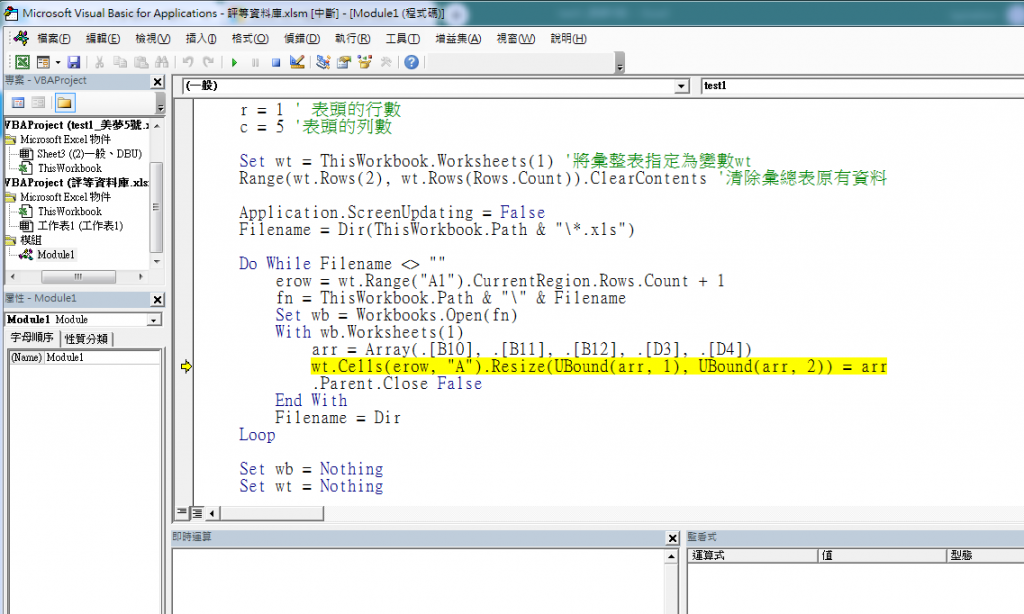#0

## 用excel vba 抓取多個excel檔特定儲存格資料 複製到新的excel特定欄位_更新

Indirect()函數確實會有這樣的弊病。

Sub HzWb()
Dim bt As Range, r As Long, c As Long
r = 1 '1 是表頭的行數
c = 7 '7 是表頭的列數
Dim wt As Worksheet
Set wt = ThisWorkbook.Worksheets(1) '將匯總表指定為變數wt
wt.Rows(r + 1 & ":1048576").ClearContents '清除匯總表中原有的資料，只保留表頭
Application.ScreenUpdating = False
Dim FileName As String, sht As Worksheet, wb As Workbook
Dim Erow As Long, fn As String, arr As Variant
FileName = Dir(ThisWorkbook.Path & "\*.xlsx")
Do While FileName <> ""
If FileName <> ThisWorkbook.Name Then '判斷檔案是否為匯總資料的活頁簿
Erow = wt.Range("A1").CurrentRegion.Rows.Count + 1 '取得匯總表中第一條空行行號
fn = ThisWorkbook.Path & "\" & FileName '將第1個要匯總的活頁簿名稱指定為變數fn
Set wb = GetObject(fn) '將變數fn 代表的活頁簿物件指定為變數wb
Set sht = wb.Worksheets(1) '將要匯總的工作表指定為變數sht
'將工作表中要匯總的記錄保存在陣列arr中
arr = sht.Range(sht.Cells(r + 1, "A"), sht.Cells(1048576, "B").End(xlUp).Offset(0, 5))
'將陣列arr 中的資料寫入工作表
wt.Cells(Erow, "A").Resize(UBound(arr, 1), UBound(arr, 2)) = arr
wb.Close False
End If
FileName = Dir '用Dir 函數取得其他檔案名，並指定為變數
Loop
Application.ScreenUpdating = True
End Sub

Sub test2()
Dim bt as Range, r as Long, c as Long
r=1 ' 表頭的行數
c=5 '表頭的列數

Dim wt as Worksheet
Set wt = ThisWorkbook.Worsheets(3) '將彙整表指定為變數wt
wt.Rows(r+1 & ":1048576").ClearContents '清除彙總表原有資料

Application.ScreenUpdating = False
Dim Filename as String, sht as Worksheet, wb as Workbook
Dim erow as Long, fn as String, arr as Variant
Filename = Dir(Thisworkbook.Path & "\*.xls")

Do While Filename <> ""
erow = wt.Range("A1").CurrentRegion.Rows.Count + 1
fn = Thisworkbook.path & "\" & Filename
set wb = getobject(fn)
set sht = wb.worksheets(1)
arr = sht.Range("D13, D12, D14, F8, F9")
wt.cells(erow, "A").resize(ubound(arr,1),ubound(arr,2)) = arr
wb.close False
end if
Filename = Dir
Loop
Application.Screenupdating = True
End Sub

### 2 個回答

0

``````Sub test2()
Dim bt as Range, r as Long, c as Long
Dim wt as Worksheet
Dim Filename as String, wb as Workbook
Dim erow as Long, fn as String, arr as Variant
r=1 ' 表頭的行數
c=5 '表頭的列數

Set wt = ThisWorkbook.Worksheets(3) '將彙整表指定為變數wt
Range(wt.Rows(2), wt.Rows(Rows.count)).ClearContents '清除彙總表原有資料

Application.ScreenUpdating = False
Filename = Dir(Thisworkbook.Path & "\*.xls")

Do While Filename <> ""
erow = wt.Range("A1").CurrentRegion.Rows.Count + 1
fn = Thisworkbook.path & "\" & Filename
Set wb = workbooks.open(fn)
With wb.worksheets(1)
arr = Array(.[D13], .[D12], .[D14], .[F8], .[F9])
wt.cells(erow, "A").resize(ubound(arr,1),ubound(arr,2)) = arr
.Parent.Close False
End With
Filename = Dir
Loop

Set wb = Nothing
Set wt = Nothing
Application.ScreenUpdating = True
End Sub
``````typo `Worsheets` -> `Worksheets`wt.Cells(erow, "A").Resize(UBound(arr)) = Application.Transpose(arr)

0

Sub HzWb()
Dim bt As Range, r As Long, c As Long
r = 1 '1 是表頭的行數
c = 7 '7 是表頭的列數
Dim wt As Worksheet
Set wt = ThisWorkbook.Worksheets(1) '將匯總表指定為變數wt
wt.Rows(r + 1 & ":1048576").ClearContents '清除匯總表中原有的資料，只保留表頭
Application.ScreenUpdating = False
Dim FileName As String, sht As Worksheet, wb As Workbook
Dim Erow As Long, fn As String, arr As Variant
FileName = Dir(ThisWorkbook.Path & "*.xlsx")
Do While FileName <> ""
If FileName <> ThisWorkbook.Name Then '判斷檔案是否為匯總資料的活頁簿
Erow = wt.Range("A1").CurrentRegion.Rows.Count + 1 '取得匯總表中第一條空行行號
fn = ThisWorkbook.Path & "" & FileName '將第1個要匯總的活頁簿名稱指定為變數fn
Set wb = GetObject(fn) '將變數fn 代表的活頁簿物件指定為變數wb
Set sht = wb.Worksheets(1) '將要匯總的工作表指定為變數sht
'將工作表中要匯總的記錄保存在陣列arr中
arr = sht.Range(sht.Cells(r + 1, "A"), sht.Cells(1048576, "B").End(xlUp).Offset(0, 5))
'將陣列arr 中的資料寫入工作表
wt.Cells(Erow, "A").Resize(UBound(arr, 1), UBound(arr, 2)) = arr
wb.Close False
End If
FileName = Dir '用Dir 函數取得其他檔案名，並指定為變數
Loop
Application.ScreenUpdating = True
End Sub

Sub test2()
Dim bt as Range, r as Long, c as Long
r=1 ' 表頭的行數
c=5 '表頭的列數

Dim wt as Worksheet
Set wt = ThisWorkbook.Worsheets(3) '將彙整表指定為變數wt
wt.Rows(r+1 & ":1048576").ClearContents '清除彙總表原有資料

Application.ScreenUpdating = False
Dim Filename as String, sht as Worksheet, wb as Workbook
Dim erow as Long, fn as String, arr as Variant
Filename = Dir(Thisworkbook.Path & "*.xls")

Do While Filename <> ""
erow = wt.Range("A1").CurrentRegion.Rows.Count + 1
fn = Thisworkbook.path & "" & Filename
set wb = getobject(fn)
set sht = wb.worksheets(1)
arr = sht.Range("D13, D12, D14, F8, F9")
wt.cells(erow, "A").resize(ubound(arr,1),ubound(arr,2)) = arr
wb.close False
end if
Filename = Dir
Loop
Application.Screenupdating = True
End Sub